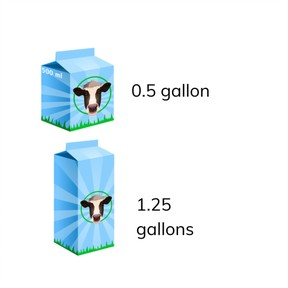Place value- compare and order decimal numbers with 1 and 2 decimal places

# Place value- compare and order decimal numbers with 1 and 2 decimal places

I can compare decimal numbers with different numbers of decimal places (1 and 2)8,000 schools use Gynzy92,000 teachers use Gynzy1,600,000 students use Gynzy

## General

Students learn to compare and set decimal numbers with 1 or 2 decimal places in numerical order. They know when a number is greater than or less than a given number.

## Relevance

Discuss with students why it is useful to be able to compare decimal numbers and set them in numerical order. Say that you can use this to determine if you have enough milk for your recipe. Which carton has enough milk?

## Introduction

Look at the numbers on the interactive whiteboard. Ask them to drag numbers with tenths to the left, and numbers with hundredths to the right. Practice how to say the numbers as you drag them to their respective circles.

## Development

Show students the decimal place value chart and use the chart to explain that you can compare decimal numbers. By comparing the tens, ones, tenths and hundredths you can determine which number is larger or smaller. Explain that you can also use the decimal place value chart to set the numbers in numerical order. Show this with an example. As a class, determine the numerical order for a set of numbers and determine which decimal number is larger or smaller. Drag the numbers to their correct locations.

Check that students can compare decimal numbers with 1 or 2 decimal places and set them in numerical order by asking the following questions/giving the following exercises:
- When is one decimal number greater than another decimal number?
- How do you know if a decimal number is greater than or less than your number
- Write a decimal number that is less than 3.1.
- Write a decimal number that is greater than 7.25.

## Guided practice

Students first practice with decimal numbers where they choose from multiple choice answers, and then they work with exercises in which they need to write the decimal number that is larger or smaller.

## Closing

Discuss with students that it is important to be able to compare decimal numbers and set them in numerical order so you can be sure you have enough of an ingredient for a recipe (like milk). Check that students know to use a decimal place value chart to help them. To check that students understood, the students must work in pairs. The first student writes two decimal numbers that are smaller than 10, one with 1 decimal place, and one with 2 decimal places, and their partner must decide which is larger or smaller. They then switch roles and repeat.

## Teaching tips

Students who have difficulty comparing decimal numbers and setting them in numerical order can practice using the decimal place value chart. By filling in the numbers in this chart they can see which number is greater or less than the other number.

### The online teaching platform for interactive whiteboards and displays in schools

• Save time building lessons

• Manage the classroom more efficiently

• Increase student engagement